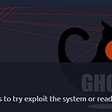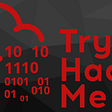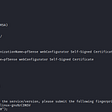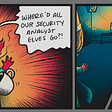# Anthem — TryHackMe

`kali@kali:~\$ sudo nmap -sS -sC -A 10.10.233.121Starting Nmap 7.80 ( https://nmap.org ) at 2020-07-13 14:16 EDTNmap scan report for 10.10.233.121Host is up (0.024s latency).Not shown: 995 closed portsPORT     STATE SERVICE       VERSION80/tcp   open  http          Microsoft HTTPAPI httpd 2.0 (SSDP/UPnP)135/tcp  open  msrpc         Microsoft Windows RPC139/tcp  open  netbios-ssn   Microsoft Windows netbios-ssn445/tcp  open  microsoft-ds?3389/tcp open  ms-wbt-server Microsoft Terminal Services| rdp-ntlm-info: |   Target_Name: WIN-LU09299160F|   NetBIOS_Domain_Name: WIN-LU09299160F|   NetBIOS_Computer_Name: WIN-LU09299160F|   DNS_Domain_Name: WIN-LU09299160F|   DNS_Computer_Name: WIN-LU09299160F|   Product_Version: 10.0.17763|_  System_Time: 2020-07-13T18:17:30+00:00| ssl-cert: Subject: commonName=WIN-LU09299160F| Not valid before: 2020-04-04T22:56:38|_Not valid after:  2020-10-04T22:56:38|_ssl-date: 2020-07-13T18:18:11+00:00; -1s from scanner time.No exact OS matches for host (If you know what OS is running on it, see https://nmap.org/submit/ ).TCP/IP fingerprint:OS:SCAN(V=7.80%E=4%D=7/13%OT=80%CT=1%CU=37572%PV=Y%DS=2%DC=T%G=Y%TM=5F0CA56OS:5%P=x86_64-pc-linux-gnu)SEQ(SP=108%GCD=1%ISR=10C%TI=I%CI=I%II=I%SS=S%TS=OS:U)OPS(O1=M508NW8NNS%O2=M508NW8NNS%O3=M508NW8%O4=M508NW8NNS%O5=M508NW8NNSOS:%O6=M508NNS)WIN(W1=FFFF%W2=FFFF%W3=FFFF%W4=FFFF%W5=FFFF%W6=FF70)ECN(R=Y%OS:DF=Y%T=80%W=FFFF%O=M508NW8NNS%CC=Y%Q=)T1(R=Y%DF=Y%T=80%S=O%A=S+%F=AS%RD=OS:0%Q=)T2(R=Y%DF=Y%T=80%W=0%S=Z%A=S%F=AR%O=%RD=0%Q=)T3(R=Y%DF=Y%T=80%W=0%SOS:=Z%A=O%F=AR%O=%RD=0%Q=)T4(R=Y%DF=Y%T=80%W=0%S=A%A=O%F=R%O=%RD=0%Q=)T5(R=OS:Y%DF=Y%T=80%W=0%S=Z%A=S+%F=AR%O=%RD=0%Q=)T6(R=Y%DF=Y%T=80%W=0%S=A%A=O%F=OS:R%O=%RD=0%Q=)T7(R=Y%DF=Y%T=80%W=0%S=Z%A=S+%F=AR%O=%RD=0%Q=)U1(R=Y%DF=N%TOS:=80%IPL=164%UN=0%RIPL=G%RID=G%RIPCK=G%RUCK=G%RUD=G)IE(R=Y%DFI=N%T=80%CD=OS:Z)Network Distance: 2 hopsService Info: OS: Windows; CPE: cpe:/o:microsoft:windowsHost script results:| smb2-security-mode: |   2.02: |_    Message signing enabled but not required| smb2-time: |   date: 2020-07-13T18:17:33|_  start_date: N/ATRACEROUTE (using port 443/tcp)HOP RTT      ADDRESS1   22.42 ms 10.9.0.12   22.55 ms 10.10.233.121OS and Service detection performed. Please report any incorrect results at https://nmap.org/submit/ .Nmap done: 1 IP address (1 host up) scanned in 76.56 seconds`
`80/tcp   open  http          Microsoft HTTPAPI httpd 2.0 (SSDP/UPnP)`
`3389/tcp open  ms-wbt-server Microsoft Terminal Services`
`nmap -n -p80 --script=http-title 10.10.69.79`

--

--

--

## More from 0xsanz

Software Developer having keen interest in Security, Privacy and Pen-testing. Certs:- Security+,PenTest+,AZ900

Love podcasts or audiobooks? Learn on the go with our new app.

## The Best Online Resources for Learning How to Code## STEM Spotlight: low-level languages, blockchain and algorithms for dummies## Clear Shot Virtual Camera in Unity 2020## GrandQuest, venture into the unknown## Run containers securely with gVisor on EKS## Creating a Geo-Redundant Path-based Web Application on Azure## Using CRDT to Resolve Conflicted Operations## 0xsanz

Software Developer having keen interest in Security, Privacy and Pen-testing. Certs:- Security+,PenTest+,AZ900

## THM: GHOST CAT## TOMGHOST — WRITE-UP## Throwback — Part 1 — pfSense## TryHackMe: [Day 14] Networking Dev(Insecure)Ops